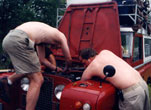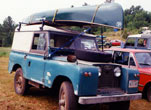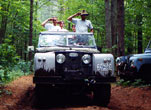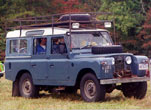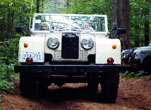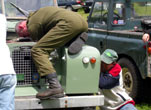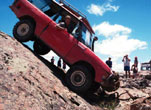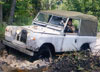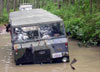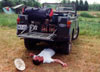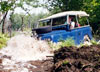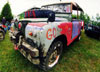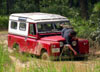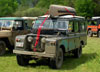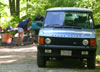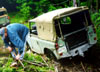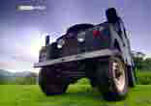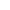Home
Introduction
Vehicle Identification
History, Production, Sales

 • Timeline • History • Decoding Chassis Numbers • Chassis Numbers By Type • Chassis Numbers By Year • IIA Chassis Number Suffixes • NAS Defender VIN Registry • Dimensions & Production • Production Data • Advertising • Land Rover VS Austin Gypsy • US Sales • North American Sales • Canadian Dealers in 73 • Trivia
Repair & Maintenance
Clubs & Parts Suppliers
Miscellaneous
What's New
Index

# CHASSIS NUMBERING BY TYPE

### Where is Your Chassis/Serial Number?

80 inch models:
The chassis number can be found on the nearside engine mounting and on the brass plate on the nearside of the bulkhead. Later vehicles had the plate positioned inside the cab.

86 & 107 models:
The chassis number can be found on the bulkhead inside the vehicle and on the right hand front spring hanger. Note: We've gotten a report of an 86" with the chassis number on the left hand *rear* spring hanger and nothing on the RH Front. So check both places.

88 & 109 models:
The chassis number can be found on the bulkhead inside the vehicle and on the right hand front spring hanger.

Series III 109" V8 (Stage I)
The chassis number can be found on the firewall in the engine bay and on the right hand front spring hanger. (Though Daryl Webb claims "LHR" whatever that means)

90, 110, 130 & Defenders:
Found in the behind Rover's left front corner of the windshield
Stamped on plate Riveted to the top of the brake pedal box in the engine compartment
Stamped on the right-hand side of the chassis forward the spring mounting turret

Range Rovers:
Found in the behind Rover's left front corner of the windshield
Stamped on the right hand side chassis member forward of the front spring mounting turret

Discoveries:
Found in the behind Rover's left front corner of the windshield
Stamped on the right hand side chassis member forward of the front spring mounting turret

### Synopsis of Chassis/Serial Numbering Scheme

1948 - 1949
Prefixed by an L or R indicating left or right-hand drive, were six or seven digits.
The first digit indicated the year:
8 = 1948-49
The second digit indicates the type:
6 = Land Rover
The third digit (applicable only to seven digit numbers) indicates the model type:
6 = Basic
7 = Station Wagon
8 = Welder

With the exception of the 866 series, whose serial numbers started at 3001, the last four digits show the serial number starting at 0001.

1950 - 1953
Prefixed by a L or R, indicating left or right-hand drive, only on the 1950 models, were eight digits.

The first digit indicates the year where:
0 = 1950
1 = 1951
2 = 1952
3 = 1953
The second digit indicates the type:
6 = Land Rover
The third digit indicates the model type:
1 = Basic
2 = Station Wagon
3 = Welder
6 = completely knocked down CKD
The fourth digit, excluding 1950 models, indicates the destination:
0 = Home market
3 = LHD Export
6 = RHD Export
With the exception of the 1950 models with five digits, the remaining four digits start at 0001 and indicate the serial number.

1954 - 1955
An eight digit numbering system

The first digit indicates the year where:
4 = 1954
5 = 1955
The second digit indicates the type:
7 = Land Rover
The third digit indicates the model type:
1 = 86 inch
2 = 107 inch
6 = 86 inch completely knocked down CKD
7 = 86 inch completely knocked down CKD
The fourth digit indicates the destination:
0 = Home market
1 = Home market
3 = LHD export
4 = LHD export
6 = RHD export
7 = RHD export
The remaining four digits start at 0001 and indicate the serial number.

1955
A nine digit digit numbering system

The first digit indicates the type:
1 = Land Rover
The second digit indicates the model:
7 = Land Rover
The third digit indicates the destination:
0 = Home market
3 = LHD export
4 = LHD CKD
6 = RHD export
7 = RHD CKD
The fourth digit indicates service period:
6 = late 1955 for service
The remaining five digits start at 00001 and indicate the serial number.

1956 - 1961
A nine digit numbering system.

The first digit on all models = 1
The second and third digits are in pairs in the following sequence indicating:
11-35 = Series I models
41-71 = Series II models
The fourth digit indicates the year:
6 = 1956
7 = 1957
8 = 1958
9 = 1959
0 = 1960
1 = 1961
The remaining five digits starting with 00001 indicate the serial number.

1962 - 1979
An eight digit chassis numbering system with suffix letters

The first, second, and third digits indicate the model and specification.
241-258,260-324 = Series IIA 88" & 109"
325-339 = Series IIB (110 Inch Forward Control)
340-354 = Series IIA 109"
5424 = Series IIA 88 Station Wagon (US Model) 259, 901-950 = Series III
951-955 & 895 = Lightweight
956-965 = 101 Inch Forward Control
355, 356, 358 = Range Rover
The remaining five digits starting with 00001 indicate the serial number.
The suffix letter indicates design modifications.
Note: The year, or model-year of manufacture have no identification number.

1980 - Date (Worldwide, except the United States)
All these years use the conventional Vehicle Identification Number (VIN)

Digits
1 Geographic Region: S = Europe
2 Country: A = United Kingdom
3 Manufacturer: L = British Leyland/Land Rover
4,5 (model)
LB = Series III, Stage I, Lightweight
LD = 90 & 110, later Defender
LH = Range Rover (mk I) (Classic)
LJ = Discovery
LN = Freelander
LP = Range Rover (mk II)
LT = Discovery, Series II
6 (wheelbase)
A = 88" & Freelander, & 100" for RR mk I
C = 109" and 1 ton
G = 100"
H = 110" & 147"
K = 127"
M = Special
R = 110"
S = Military 110"
V = 92.3"
7 (body type)
A = Truck Cab, Soft Top or Hard Top (Utility body)
B = 3 door Station Wagon, or Freelander 5 door hardtop
E = 2 door Crew Cab
F = 4 door Crew Cab
H = High Capacity pick-up (Some had an A instead of the H)
M = 5 door Station Wagon & 7 Door SW
R = Monteverdi Range Rover
8 (engine)
B = 2.5l, 4 cylinder, Turbo diesel
C = 2.5l, 4 cylinder, diesel
D = 2.5l, 4 cylinder, petrol
F = 200 Tdi & 300 Tdi (2.5 Liter, 4 cylinder, Turbor Diesels)
G = 2.25l diesel & Freelander V6
H = 2.25l petrol
M = 3.9 liter V8 (injection)
P = 2.6l 6 cylinder, petrol
V = 3.5l V8 petrol (carburettor)
Y = 2 liter T16 petrol
Z = 3.9 liter Izuzu diesel
8 = Td5
9 (steering/transmission)
1 = RHD, 4 speed
2 = LHD, 4 speed
3 = RHD, automatic
4 = LHD, automatic
5 = RHD, 4 speed & overdrive
6 = LHD, 4 speed & overdrive
7 = RHD, 5 speed
8 = LHD, 5 speed
10 (model variant/model year?)
A = up to 1983-1984
B = 1985-1987
E = 1988
F = 1989
G = 1990
H = 1991
J = 1992
K = 1993
L = 1994
M = 1995
N = 1996
? = 1997
? = 1998
W = 1999
? = 2000
1 = 2001
2 = 2002
11 (assembly location)
A = Solihull
F = CKD
V = South Africa
12-17 (serial number)
1987 - Date (USA)
The USA has slightly different Vehicle Identification Number (VIN) from the world. Digits 1,2,3 are set by the SAE (Society of Automotive Engineers) for each automotive company

Digits
1 Country: S = UK
2 Manufacturer: A = British Leyland/Rover
3 Vehicle Make: L = Land Rover
4 Line (model)
D = 90 & 110
H = Range Rover Classic
J = Discovery
N = Freelander
P = Range Rover (mk2)
T = Discovery, Series II
5 Series (Emissions and wheelbase)
C = LWB (108") Range Rover Classic--must be a 49 State Emmissions
E = 50 State Emisssions: NAS Range Rover, mkII (100")
H = NAS 110"
N = 50 State Emissions: NAS Discovery (100")
V = 49 State Emissions: NAS 90" or NAS Range Rover, mkII (100")
50 State Emission for NAS Range Rover Classic (100" & 108") X = 50 State Emissions (California): NAS 90"
Y = 49 State Emissions: NAS Discovery (100") & Freelander
6 Body Type
1 = closed (hard top), 5 door
2 = open (soft top), 2 door. NAS D90s & Freelander
3 = closed (hardtop), 3 door. NAS D90 Station Wagon
7 Engine Type
1 = 3.5 L V8
2 = 3.9 L V8 and 4.0 L V8 (OBD II) or Freelander V6
3 = 4.2 L V8 (LWB Range Rover Classic
4 = 4.6 L V8
8 Steering/transmission and Gross Vehicle Weight class)
2 = Freelander automatic
3 = Canadian D110 and 6000 lb GVW
4 = LHD 4 speed automatic and 6000 lb GVW
8 = LHD 5 speed manual and 6000 lb GVW
9 Checksum for the other 16 digits
May be any digit from 0 to 9 or X.
1. A numberic value is assigned to each letter in the VIN
1. A,J
2. B,K,S
3. C,L,T
4. D,M,U
5. E,N,V
6. F,W
7. G,P,X
8. H,Y
9. R,Z
2. Each position of the VIN is given a weight factor
1=8, 2=7, ... , 7=2, 8=10, 10=9, ... , 17=2
3. Multiply each digit by it's weight factor and sum for positions 1 to 8 and 10 to 17.
4. Divided by 11 and find the remainder. The remainder is the 9th digit (with and X being used if the remainder is 10)
Example: a 1996 Range Rover Classic (SALHE124XSA660000)
```
VIN:        S  A  L  H  E  1  2  4  X  S  A  6  6  0  0  0  0
number:     2  1  3  8  5  1  2  4  *  2  1  6  6  0  0  0  0
Weight:     7  6  5  4  3  2  1 10     9  8  7  6  5  4  3  2
Multiply:  14  6 15 32 15  2  2 40    18  8 42 36  0  0  0  0
Add:       14 +6+15+32+15 +2 +2+40   +18 +8+42+36+ 0+ 0+ 0+ 0 = 230
Divide:    230/11= 20 remainder 10.  So the 9th Digit is an X (as shown)
```
10 Year of Manufacture
J=1988
K=1989
L=1990
M=1991
N=1992
P=1993
R=1994
S=1995
T=1996
V=1997
X=1998
Y=1999
Z=2000
1=2001
2=2002
11 (assembly location)
A = Solihull
F = CKD
12-17 (serial number)
See Specific vehicle FAQs for sequences